# Todd-Coxeter algorithm and uniform polytopes

This project renders 3d/4d uniform polytopes using Python and POV-Ray, the code is on github.

Requirements: numpy and the free raytracer POV-Ray.

# Examples

All images and videos below are created with this program. The data of the polytopes are computed in Python and then exported to POV-Ray for rendering. There are also some tricky CSG computations in the POV-Ray part, but that's not the main focus of this document. The POV-Ray code is only for illustrating the procedure, the default settings (i.e. camera, lights, textures) may not work well for all polytopes. You can modify them to render your own scenes.

• All Platonic solids and Archimedean solids, prims and antiprisms, for example the snub dodecahedron:

• All Kepler-Poinsot solids, for example the great icosahedron:

• All uniform 4d polytopes (except the grand antiprism, which is non-Wythoffian), for example my github favicon, the runcinated 120-cell: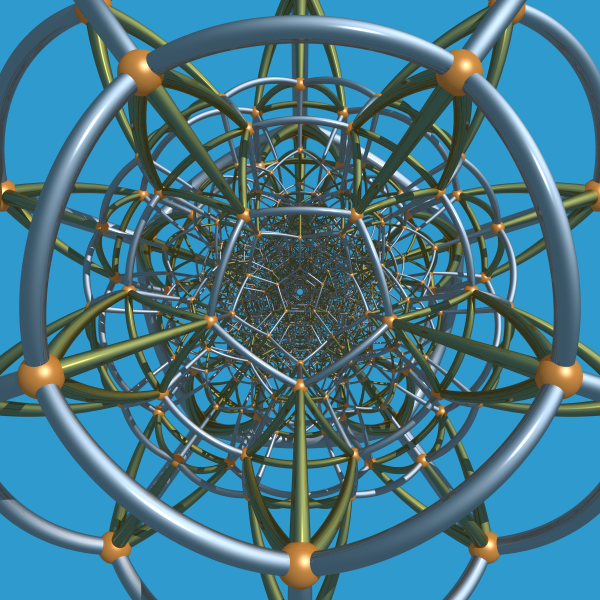• 5-cell: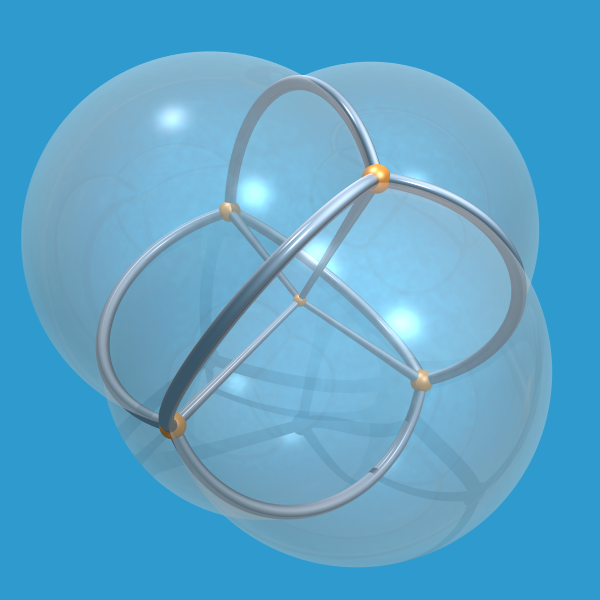• 4d cube: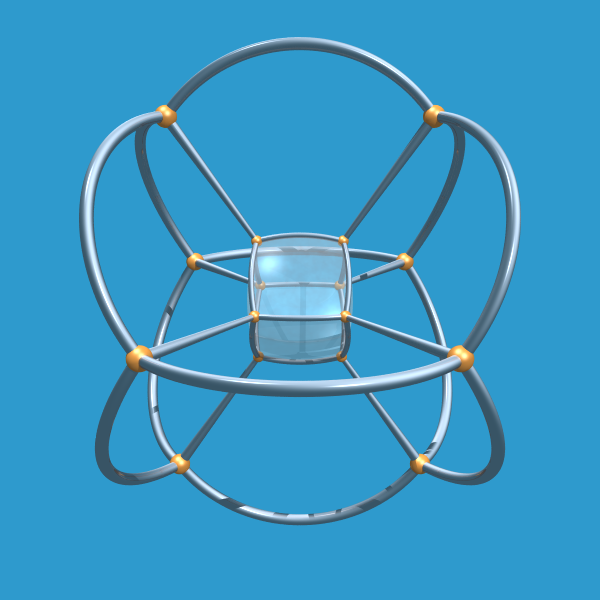• 600-cell: (you can render the bubble faces and choose which of them are shown)•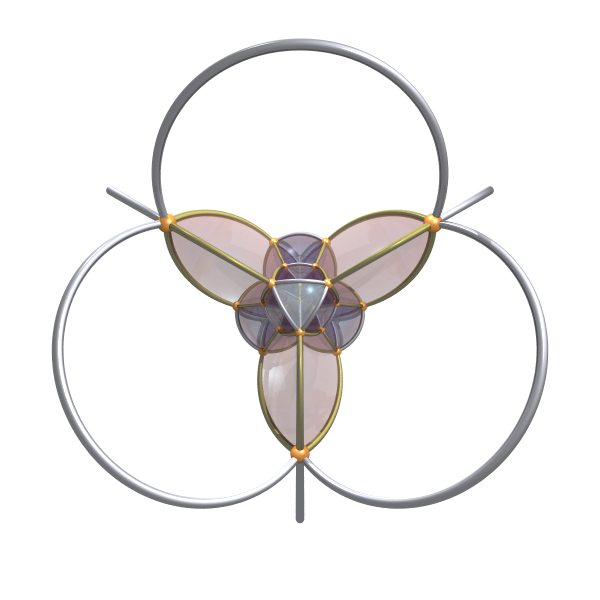• You can also render uniform star 4d polytopes, for example the grand stellated 120-cell:

and its rectified version (rendered in a curved fashion):

•• And finally, uniform 5D polytopes like 5-cube:

# What are these examples about?

Roughly speaking, all polytopes shown above are convex/non-convex, uniform polytopes in 3d/4d Euclidean spaces. Here we note the keywords: convex/non-convex, Euclidean and uniform.

"converx" is quite easy to understand, it means that any line segment joins two points on the polytope lies entirely in the enclosure of the polytope. Platonic solids, Archimedean solids and Catalan solids are all convex, whereas Kepler-Poinsot solids and star polychoron are all non-convex.

In the 3d Euclidean space there are 18 different convex uniform polytopes (if we exclude the two infinite classes prisms and antiprisms) and 57 different non-convex uniform polytopes. Currently this program can only render the convex ones and a few non-convex ones, I need to figure out the right way to make it work for all of them in the future.

"Euclidean" is also easy to understand, it's emphasized here because we also have uniform polytopes in other metric spaces (for example the hyperbolic metric) which bends the space and makes the polytopes look like "deformed". The most famous example in this kind is the logo "Spikey" of mathematica, it's based on the dodecahedron in hyperbolic 3-space:The word "uniform" requires some math subtleties. Roughly it says

1. all the vertices are the same.
2. all the faces are regular polygons.
3. all the cells are uniform polyhedron (an uniform polyhedra is a polyhedra satisfies 1 and 2).

To explain what is "the same", we need some terms from group theory: it means that the symmetry group $G$ of the polytope acts transitively on the set of vertices, that is, for any pair of vertices $u,v$, there is some $g\in G$ that transforms $v$ to $u$: $g\cdot u=v$.

In the above examples the polytopes are colored so that all vertices/edges/faces that are in the same orbit under the action of the symmetry group have the same color.

# How to compute the data of an uniform polytope

Though these polytopes look quite different in appearance, all of them can be constructed in an uniform apprach called the Wythonff construction (also named the kaleidoscope construction). In principal it's the same with the way how a kaleidoscope works: we place some reflection planes (called mirrors) in the space, where all these mirrors pass through the origin and the angles between them are carefully chosen (these angles must be of the form $\pi-\pi/p$ for some rational $p$). These mirrors partitioned the space into "rooms". Choose any room (called the fundamental domain) and an initial vertex $v_0$ in it, we then repeatly reflect $v_0$ about the mirrors to get the set of virtual images. All virtual images together with $v_0$ form the vertices of our polytope. If $v_1$ is the virtual image of the initial point $v_0$ about the $i$-th mirror then $(v_0,v_1)$ form an edge of type $i$, we can use the symmetry group to populate it to other edges in this orbit and color them with the $i$-th color in our palette. Also if $v_0$ is firstly reflected about mirror $i$ and then reflected about mirrot $j$, then since the composition of two reflections is a rotation, $v_0$ is rotated about the center of some face $f$ by an angle of $2\pi/m$ (assume the angle between mirror $i$ and mirror $j$ is $\pi-\pi/m$). We can recover $f$ by applying this rotation $m$ times and again use the symmetry group to populate it to other faces.

To implement the above strategy into a pratical program we have two main problems to solve:

1. For a given convex unifom polytope, how should we place the mirrors and choose the initial vertex $v_0$?
2. When the mirrors and $v_0$ are ready for use, how can we compute all virtual images of $v_0$?

The answer to the first question is called Coxeter-Dynkin diagram, which is a labelled undirected graph that encodes all information we will need. Each uniform polytope has a corresponding Coxeter-Dynkin diagram represents it, but different Coxeter-Dynkin diagrams may represent the same polytope.

For example the Coxeter-Dynkin diagram of the cube isWe now explain this diagram in details:

In a Coxeter-Dynkin diagram each node represents a mirror in the kaleidoscope. The above diagram has three nodes, hence there are three mirrors in the case of cube. We label the corresponding mirrors from left to right as $m_0,m_1,m_2$. For a pair of nodes the labelled edge between them encodes the angle between their mirrors:

1. Two nodes are not connected iff the angle between their mirrors is exactly $\pi/2$.
2. Two nodes are connected by an unlabelled edge iff the angle between their mirrors is exactly $\pi-\pi/3$.
3. Two nodes are connected by an edge labelled with a rational number $m>2$ and $m\ne3$ iff the angle between their mirrors is $\pi-\pi/m$.

Also we use "circled" nodes to indicate which mirrors are "active": a mirror is called active if and only if it does not contain the initial vertex $v_0$ in it, or in other words, if and only if reflecting $v_0$ about this mirror gives a virtual image.

So in the above example we have $\langle m_0,m_1\rangle=\pi-\pi/4$, $\langle m_1,m_2\rangle=\pi-\pi/3$, $\langle m_0,m_2\rangle=\pi/2$. $m_0$ is active but $m_1$ and $m_2$ are not.

Hence we can place these three mirrors in the 3d space as follows: (we use $n_i$ to denote the unit normal vector of $m_i$)

1. The normal of $m_0$ can be arbitrary, for example $n_0=(1,0,0)$.
2. The angle between $n_1$ and $n_0$ is $3\pi/4$, we can simply choose $n_1$ to be $n_1=(\cos\dfrac{3\pi}{4}, \sin\dfrac{3\pi}{4}, 0)$.
3. The normal of $m_2$ is perpendicular to $n_0$, so $n_2$ has form $(0,y_3,z_3)$. By $\langle n_1,n_2\rangle=2\pi/3$ we have $y_3\sin\dfrac{3\pi}{4}=\cos\dfrac{2\pi}{3}$ and $z_3=\sqrt{1−y_3^2}$. Solve these two equations to get $y_3,z_3$.

To choose an initial vertex $v_0$ which lies on $m_1,m_2$ but not on $m_0$, we can let $v_0$ has distance 1 to $m_0$ and distance 0 to $m_1$ and $m_2$:

\begin{align*}\langle v_0, n_0\rangle=1,\\\langle v_0, n_1\rangle=0,\\\langle v_0, n_2\rangle=0.\\\end{align*}

Simply solve this linear system to get $v_0$.

I mentioned before that the angles between the mirrors must be carefully chosen to ensure that $v_0$ and its virtual images form the vertices of an uniform polytope, this restricts us to only finitely many choices, you can refer to this wiki page for the complete list.

The answer to the second question is called Todd-Coxeter algorithm, I'll discuss it in the next section.

# Finitely presented groups and Todd-Coxeter algorithm

How can one get all virtual images of the initial vertex $v_0$ about all the mirrors? An obvious (and also crude) way to do this is to simply repeatly reflect $v_0$ abut those mirrors, and after each reflection compare the result with the set of virtual images obtained so far (within a predefined rounding error bound) until no more new virtual images can occcur. This approach is easy to program and has the advantage that it's almost the unique choice to implement Wythoff constrution in shaders, for example see this great shadertoy program by Matt Zucker. But this approach is ugly from a mathematician's aesthetic: it did not use the plenty of symmetries inherent in the polytope!

This program takes another "symbolic computation" approach by solving the coset enumeration problem in the symmetry group. It has the advantage that one can get all exact information of the polytope without using any rounding errors nor approximating procedures. The price paid here is that the math invovled is a bit complicated (hence also the code) and the reader needs to have some preliminary knowledge of group theory to understand it.

Firstly let's recall the orbit-stabilizer theorem from group theory:

Theorem: If a group $G$ acts transitively on a set $S$ and assume for some $x\in S$ the stabilizing subgroup of $x$ is $H\leq G$, then there is a one-to-one correspondence between $S$ and the right cosets in $G/H$: $x\cdot g\to Hg$.

Note the action of $G$ on $S$ is written as "applying on the right", this is mainly for programming convenience and has no significant difference with applying on the left.

The theorem tells us that if a group $G$ acts transitively on a set $S$ and for some $x\in S$ we know its stabilizing subgroup in $G$ is $H$, then we can recover the whole orbit $S$ by applying a set of coset representatives of $G/H$ on $x$.

So for a given uniform polytope $P$, we can compute all its vertices as follows:

1. Get a presentation of its symmetry group $G$ and the coordinates of an initial vertex $v_0$ from the Coxeter-Dynkin diagram.
2. Get a presentation of $v_0$'s stabilizing subgroup $H$ and compute a set of right coset representatives of $G/H$.
3. Apply these representatives on $v_0$ to get all vertices.

Again we use the cube as an example to show this procedure: recall the Coxeter-Dynkin diagram of the cube isThe mirrors are $m_0,m_1,m_2$, let their normals be $n_0,n_1,n_2$ which are all unit vectors, denote the reflections about these mirrors as $\rho_0,\rho_1,\rho_2$, the matrix of $\rho_i$ is $M_i=I - 2n_in_i^T$ (see Householder transformation).

The symmetry group $G$ of the cube is generated by the above three "generator reflections" $\rho_0,\rho_1,\rho_2$, a presentation is

$G = \langle\rho_0,\rho_1,\rho_2\ |\ \rho_0^2=\rho_1^2=\rho_2^2=(\rho_0\rho_1)^4=(\rho_1\rho_2)^3=(\rho_0\rho_2)^2=1\rangle.$

This is because a reflection is always involutionay, i.e. has order 2, and since $\rho_0, \rho_1$ are two reflections with angle $3\pi/4$ between their mirrors, $\rho_0\rho_1$ is a rotation about the line of intersection of their mirrors with angle $3\pi/2$, hence $(\rho_0\rho_1)^4=1$. Similarily one has the relations for $\rho_1\rho_2$ and $\rho_0\rho_2$.

Note: You may ask if we are missing some "hidden" relations here, this is a good question. For convex polytopes the answer is no, but for some star polytopes the answer is yes, we have to add some extra relations to make the procedure work fine. A remarkable difference is that in convex cases the symmetry group always map the interior of the fundamental domain to another disjoint one, whereas in the cases of star polytopes the fundamental domain maybe covered by other domains several times. See Vinberg's paper "Discrete linear groups generated by reflections".

One can use Todd-Coxeter algorithm (will be explained later) to compute a complete list of the elements in $G$ (48 in total):

$\begin{array}{lll}e&\rho_{0}&\rho_{0}\rho_{1}\\\rho_{0}\rho_{1}\rho_{0}&\rho_{0}\rho_{1}\rho_{0}\rho_{1}&\rho_{1}\rho_{0}\rho_{1}\\\rho_{1}\rho_{0}&\rho_{1}&\rho_{0}\rho_{2}\\\rho_{2}&\rho_{1}\rho_{2}&\rho_{1}\rho_{2}\rho_{1}\\\rho_{2}\rho_{1}&\rho_{0}\rho_{1}\rho_{2}&\rho_{0}\rho_{1}\rho_{2}\rho_{1}\\\rho_{0}\rho_{2}\rho_{1}&\rho_{0}\rho_{1}\rho_{0}\rho_{2}&\rho_{0}\rho_{1}\rho_{0}\rho_{1}\rho_{2}\\\rho_{0}\rho_{1}\rho_{0}\rho_{1}\rho_{2}\rho_{1}&\rho_{0}\rho_{1}\rho_{0}\rho_{2}\rho_{1}&\rho_{1}\rho_{0}\rho_{1}\rho_{2}\\\rho_{1}\rho_{0}\rho_{2}&\rho_{1}\rho_{0}\rho_{2}\rho_{1}&\rho_{1}\rho_{0}\rho_{1}\rho_{2}\rho_{1}\\\rho_{2}\rho_{1}\rho_{0}&\rho_{2}\rho_{1}\rho_{0}\rho_{1}&\rho_{0}\rho_{2}\rho_{1}\rho_{0}\rho_{1}\\\rho_{0}\rho_{2}\rho_{1}\rho_{0}&\rho_{1}\rho_{0}\rho_{2}\rho_{1}\rho_{0}&\rho_{1}\rho_{0}\rho_{2}\rho_{1}\rho_{0}\rho_{1}\\\rho_{1}\rho_{2}\rho_{1}\rho_{0}\rho_{1}&\rho_{1}\rho_{2}\rho_{1}\rho_{0}&\rho_{0}\rho_{1}\rho_{0}\rho_{2}\rho_{1}\rho_{0}\\\rho_{0}\rho_{1}\rho_{0}\rho_{2}\rho_{1}\rho_{0}\rho_{1}&\rho_{0}\rho_{1}\rho_{2}\rho_{1}\rho_{0}\rho_{1}&\rho_{0}\rho_{1}\rho_{2}\rho_{1}\rho_{0}\\\rho_{1}\rho_{0}\rho_{1}\rho_{2}\rho_{1}\rho_{0}&\rho_{1}\rho_{0}\rho_{1}\rho_{2}\rho_{1}\rho_{0}\rho_{1}&\rho_{0}\rho_{1}\rho_{0}\rho_{1}\rho_{2}\rho_{1}\rho_{0}\rho_{1}\\\rho_{0}\rho_{1}\rho_{0}\rho_{1}\rho_{2}\rho_{1}\rho_{0}&\rho_{2}\rho_{1}\rho_{0}\rho_{1}\rho_{2}&\rho_{1}\rho_{2}\rho_{1}\rho_{0}\rho_{1}\rho_{2}\\\rho_{0}\rho_{2}\rho_{1}\rho_{0}\rho_{1}\rho_{2}&\rho_{0}\rho_{1}\rho_{2}\rho_{1}\rho_{0}\rho_{1}\rho_{2}&\rho_{1}\rho_{0}\rho_{2}\rho_{1}\rho_{0}\rho_{1}\rho_{2}\\\rho_{1}\rho_{0}\rho_{1}\rho_{2}\rho_{1}\rho_{0}\rho_{1}\rho_{2}&\rho_{0}\rho_{1}\rho_{0}\rho_{2}\rho_{1}\rho_{0}\rho_{1}\rho_{2}&\rho_{0}\rho_{1}\rho_{0}\rho_{1}\rho_{2}\rho_{1}\rho_{0}\rho_{1}\rho_{2}\end{array}$

Since in the Coxeter-Dynkin diagram only the mirror $m_0$ is active, i.e. the initial vertex $v_0$ is on $m_1,m_2$ but not on $m_0$, both $\rho_1$ and $\rho_2$ map $v_0$ to itself, while $\rho_0$ maps $v_0$ to its virtual image about $m_0$, hence the stabilizing subgroup of $v_0$ is

$H=\langle \rho_1, \rho_2\ |\ \rho_1^2=\rho_2^2=(\rho_1\rho_2)^3=e\rangle.$

Note: You may ask that we can only say the subgroup generated by $\{\rho_0,\rho_1\}$ is contained in the stabilizing subgroup of $v_0$ and may not be exactly equal to it. Indeed it does. This is a property of Coxeter groups: in the geometric realization of a Coxeter group $W$ (that is, represent $W$ as a set of reflections about hyperplanes in $\mathbb{R}^n$), for any point $v$ in the fundamental domain, the stabilizing subgroup of $v$ is a standard parabolic subgroup generated by those simple reflections whose hyperplanes contain $v$. This obvious geometry intuition requires a quite non-trivial proof, see Humphreys's book "reflection groups and Coxeter groups", chapter 1.

Obviously $H$ is the dihedral group $D_3$ hence $|H|=6$ and $|G/H|=8$. Again by applying Todd-Coxeter algorithm one can get a list of coset representatives of $G/H$:

$\begin{array}{llll}e&\rho_{0}&\rho_{0}\rho_{1}&\rho_{0}\rho_{1}\rho_{0}\\\rho_{0}\rho_{1}\rho_{2}&\rho_{0}\rho_{1}\rho_{0}\rho_{2}&\rho_{0}\rho_{1}\rho_{0}\rho_{2}\rho_{1}&\rho_{0}\rho_{1}\rho_{0}\rho_{2}\rho_{1}\rho_{0}\end{array}$

Apply them on $v_0$ one gets the 8 vertices of the cube. For example the action of $\rho_0\rho_1$ on $v_0$ is given by

$v_0(\rho_0\rho_1)=(v_0\rho_0)\rho_1=(v_0M_0)\rho_1=v_0M_0M_1.$

Here $v_0$ is written as a row vector since all $M_i$'s are symmetric matrices.

The above procedure also works for edges and faces. For example if we want to find all edges of type $i$, we can go as follows:

1. Check if $v_0$ is on the mirror $m_i$. If the answer is yes, then the reflection $\rho_i$ fixes $v_0$ and there are no edges of type $i$, else let $v_1=\rho_i(v_0)$, then $(v_0,v_1)$ form an edge $e$ of type $i$.
2. A symmetry fixes $e$ if and only if it fixes the middle point $p$ of $e$, hence the stabilizing suggroup of $e$ is the same with the stabilizing subgroup of $p$, say this subgroup is $H$. From the comment above we know $H$ is generated by those simple reflections with their mirrors contain $p$. It's easy to see that such mirrors are either $m_i$, or those contain $v_0$ and are orthogonal to $m_i$ (so $e$ lies entirely in such mirrors), hence $H$ is generated by $\rho_i$ and those simple reflections that fix $v_0$ and commute with $\rho_i$. In the cube example $H=\langle \rho_0,\rho_2\rangle$, so $|H|=4$ and $|G/H|=12$, we have verified the fact that cube has 12 edges.
3. Find a set of coset representatives of $G/H$ and apply them on $e$ to get all edges of type $i$.

Finding all faces requires more subtle work but the principals are still the same. For a pair $i\ne j$, if at least one of $m_i,m_j$ is active then the rotation $r_{ij}=\rho_i\rho_j$ generates a face $f$ of type $(i,j)$ and $f$ is invariant under $\rho_i$ and $\rho_j$. Here we need to becareful of the case that $v_0$ is on exactly one of the two mirrors and they are perpendicular. Anyway the stabilizing subgroup is generated by $\rho_i,\rho_j$ plus those generators that fix $v_0$ and commute with both $\rho_i$ and $\rho_j$. Again we compute a set of coset representatives of $G/H$ and apply them on $f$ to get all faces of type $(i,j)$.

Now the key step reduces to computing a set of coset representatives of $G/H$ for a finitely presented group $G$ and its subgroup $H$, this is exactly what Todd-Coxeter algorithm does.

Todd-Coxeter algorithm is very much like playing a sudoku game in which the table to complete is a dynamically growing 2d array $T$, the rows of $T$ are labelled by the right cosets in $G/H$ and the columns of $T$ are labelled by the generators of $G$. $T[i][j]$ records the right coset obtained by multiplicating the $j$-th generator on the right of the $i$-th coset. The algorithm repeatly uses the defining relations in the presentation of $G$ and $H$ as guidelines to find new cosets and fill their corresponding entries in $T$. The game is over once all entries in $T$ are filled, the coset in each entry has a row in $T$ and all relations are satisfied by each row. Such $T$ obtained is nothing but the adjacent matrix of the schreier graph of $G/H$ and one can easily get a complete list of word representations for all cosets in $G/H$.

For a detailed treatment of Todd-Coxeter algorithm the reader should refer to (also referred as HCGT in this document)

Chapter 5, Handbook of Computational Group Theory, Holt, D., Eick, B., O'Brien, E.

I will show in the below how the algorithm goes using the cube again as example:

Example: let $G$ be the symmetric group of the cube: \begin{align*} G = \langle\rho_0,\rho_1,\rho_2\ |\ \rho_0^2&=\rho_1^2=\rho_2^2=(\rho_0\rho_1)^4=(\rho_1\rho_2)^3\\ &=(\rho_0\rho_2)^2=1\rangle.\end{align*} and subgroup $H=\langle \rho_1, \rho_2\rangle$. Find a set of coset representatives of $G/H$.

Let's list down all known relations for this sudoku game first:

Our known relations:

1. For each generator word $w$ of $H$ it holds $Hw=H$, i.e. $H\rho_1=H$ and $H\rho_2=H$.
2. For any coset $K$ and any generator relation $r$ of $G$ it holds $Kr=K$, i.e. $K\rho_i^2=K,i=0,1,2$, and $K(\rho_0\rho_1)^4=K(\rho_1\rho_2)^3=K(\rho_0\rho_2)^2=K.$

These relations can be stored in two lists, one for the relations in $H$ and one for the relations in $G$, each relation can be further stored as an array of int type.

The first list stores the generator words of $H$:

1. (1,) // $\rho_1$
2. (2,) // $\rho_2$

The second list stores the defining relations of $G$:

1. (0, 0) // $\rho_0^2=1$
2. (1, 1) // $\rho_1^2=1$
3. (2, 2) // $\rho_2^2=1$
4. (0, 1, 0, 1, 0, 1, 0, 1) // ($\rho_0\rho_1)^4=1$
5. (1, 2, 1, 2, 1, 2) // ($\rho_1\rho_2)^3=1$
6. (0, 2, 0, 2) // ($\rho_0\rho_2)^2=1$

The relations are numbered from 0-7 so later we can refer to them conveniently.

Note: when $G$ is not represented in the form of a Coxeter group (for example the case of snub polytopes) we should also take account of the inverse of the generators and they too occupy their columns in $T$, so the actual number of columns in $T$ is 2 $\times$ (number of generators). But for Coxeter groups all generators are involutions so there is no need to insert the columns for their inverse.

Initially the table $T$ has only one row in it:

$\phantom{}$ $\rho_0$ $\rho_1$ $\rho_2$
$H_0$ $\phantom{}$ $\phantom{}$ $\phantom{}$

where $H_0=H$.

The algorithm firstly checks if $H_0$ satisfies all relations in the first list (once this is done the first list can be discarded) and then scans all cosets of $T$ from top to bottom and make sure the current coset satisfies all relations in the second list. In this process new cosets are defined and their rows are appended at the end of $T$, and be careful it may happen that some cosets in the table indeed represent the same coset.

Let's begin by scanning $H_0$ and check the relations in the first list are satisfied by it:

(1). For relation 0 we have $H_0\rho_1=H_0$, i.e. $T=0$, for relation 1 we have $H_0\rho_2=H_0$, i.e. $T=0$. $T$ becomes

$\phantom{}$ $\rho_0$ $\rho_1$ $\rho_2$
$H_0$ $\phantom{}$ 0 0

Now $H_0$ satisfies all relations in the first list so this list can be discarded. From now on we only check relations in the second list.

we move on to check the relations in the second list are satisfied by $H_0$:

(2). Relation 2 says $H_0\rho_0^2=H_0$, since we do not know $H_0\rho_0$ yet, we define it to be $H_1$, fill 1 in its entry $T$ and also append a new row for $H_1$:

$\phantom{}$ $\rho_0$ $\rho_1$ $\rho_2$
$H_0$ 1 0 0
$H_1$ 0 $\phantom{}$ $\phantom{}$

Note each time when we define or find $H_i\rho_j=H_k$ for some $i,j,k$ we automatically get the "dual" relation $H_k\rho_j=H_i$, so we always fill in a pair of entries $T[i][j]=k$ and $T[k][j]=i$ at a time.

(3). Relations 3, 4 are already satisfied, continue.

(4). Relation 5 says $H_0\rho_0\rho_1\rho_0\rho_1\rho_0\rho_1\rho_0\rho_1=H_0$. We already know $H_0\rho_0=H_1$ but $H_1\rho_1$ is unknown, so we define it to be $H_2$, fill in the two entries and append a new row for $H_2$:

$\phantom{}$ $\rho_0$ $\rho_1$ $\rho_2$
$H_0$ 1 0 0
$H_1$ 0 2 $\phantom{}$
$H_2$ $\phantom{}$ 1 $\phantom{}$

$H_2\rho_0$ is again unknown, so we define $H_2\rho_0=H_3$ and $T$ becomes

$\rho_0$ $\rho_1$ $\rho_2$
$H_0$ 1 0 0
$H_1$ 0 2 $\phantom{}$
$H_2$ 3 1 $\phantom{}$
$H_3$ 2 $\phantom{}$ $\phantom{}$

Continuing the scanning we find $H_3\rho_1$ is again unknown, you may think that we can define it to be a new coset $H_4$ as we did before and then move on. This strategy works in principal but in practice it creates many redundant cosets and makes $T$ grow very rapidly. Instead we scan the relation "backward" to try to fill the gaps without defining new cosets. Remember we have scanned from the left to the following position: $H_0\rho_0\rho_1\rho_0(=H_3)\rho_1\rho_0\rho_1\rho_0\rho_1=H_0.$ Scanning the above relation from right to left we have $H_0\rho_1\rho_0\rho_1\rho_0=H_3$, that is, $H_0\rho_0\rho_1\rho_0(=H_3)\rho_1 =H_0\rho_1\rho_0\rho_1\rho_0=H_3.$ Hence $H_3\rho_1=H_3$ and we have deduced the coset $H_3\rho_1$ instead of defining it to be a new coset. We call this a deduction according to the book HCGT, $T$ now becomes

$\phantom{}$ $\rho_0$ $\rho_1$ $\rho_2$
$H_0$ 1 0 0
$H_1$ 0 2 $\phantom{}$
$H_2$ 3 1 $\phantom{}$
$H_3$ 2 3 $\phantom{}$

So in the actual program we always scan a relation from both ends and define new cosets if necessary until they meet.

(5). Relation 6 is already satisfied, continue.

(6). Relation 7 says $H_0\rho_0\rho_2\rho_0\rho_2=H_0$, scanning from both ends gives $H_0\rho_0(=H_1)\rho_2=H_0\rho_2\rho_0=H_1,$ hence $H_1\rho_2=H_1$ and we got another deduction. $T$ now becomes

$\phantom{}$ $\rho_0$ $\rho_1$ $\rho_2$
$H_0$ 1 0 0
$H_1$ 0 2 1
$H_2$ 3 1 $\phantom{}$
$H_3$ 2 3 $\phantom{}$

Now $H_0$ satisfies all relations in the two lists so the scanning of the first row is completed. We move on and begin the scanning of the row for $H_1$. Note the first list is now discarded, we will only check relations 2-7.

(1). Relations 2, 3, 4, 5 are already satisfied, continue.

(2). Relation 6 says $H_1\rho_1\rho_2\rho_1\rho_2\rho_1\rho_2=H_1$, in which $H_1\rho_1=H_2$ is known but $H_2\rho_2$ is unknown. The backward scanning also get stuck here: $H_1\rho_1(=H_2)\rho_2\rho_1=H_1\rho_2\rho_1\rho_2=H_2\rho_2.$ So we define $H_2\rho_2=H_4$, then $H_4\rho_1=H_4$ and $T$ becomes

$\rho_0$ $\rho_1$ $\rho_2$
$H_0$ 1 0 0
$H_1$ 0 2 1
$H_2$ 3 1 4
$H_3$ 2 3 $\phantom{}$
$H_4$ $\phantom{}$ 4 2
1. One can check relation 7 is already satisfied so the scanning of $H_1$ is completed and we move on to scan the row for $H_2$.

I'll leave the scanning of $H_2,H_3,H_4,H_5$ to you as easy exercises. After $H_2$ is completed your $T$ should be

$\rho_0$ $\rho_1$ $\rho_2$
$H_0$ 1 0 0
$H_1$ 0 2 1
$H_2$ 3 1 4
$H_3$ 2 3 5
$H_4$ 5 4 2
$H_5$ 4 $\phantom{}$ 3

After $H_3$ is completed your $T$ should be

$\rho_0$ $\rho_1$ $\rho_2$
$H_0$ 1 0 0
$H_1$ 0 2 1
$H_2$ 3 1 4
$H_3$ 2 3 5
$H_4$ 5 4 2
$H_5$ 4 6 3
$H_6$ $\phantom{}$ 5 6

After $H_4$ is completed your $T$ should be

$\rho_0$ $\rho_1$ $\rho_2$
$H_0$ 1 0 0
$H_1$ 0 2 1
$H_2$ 3 1 4
$H_3$ 2 3 5
$H_4$ 5 4 2
$H_5$ 4 6 3
$H_6$ 7 5 6
$H_7$ 6 7 $\phantom{}$

And the scanning of $H_5$ gives no more information.

When scanning $H_6$ we found that relations 2-6 are already satisfied, from relation 7 $H_6\rho_0\rho_2\rho_0\rho_2=H_6$ we get a deduction $H_7\rho_2=H_7$ and $T$ becomes

$\rho_0$ $\rho_1$ $\rho_2$
$H_0$ 1 0 0
$H_1$ 0 2 1
$H_2$ 3 1 4
$H_3$ 2 3 5
$H_4$ 5 4 2
$H_5$ 4 6 3
$H_6$ 7 5 6
$H_7$ 6 7 7

One can check that $H_7$ satisfies all relations in the second list, so no more cosets can be found and the game is over.

Using breadth-first search one can get the multiplication relations between these cosets:

$\begin{array}{l}H_0 = H_0\cdot e,\\ H_1=H_0\cdot\rho_0,\\H_2=H_1\cdot\rho_1=H_0\cdot\rho_0\rho_1,\\H_3=H_2\cdot\rho_0=H_0\cdot\rho_0\rho_1\rho_0,\\ H_4=H_2\cdot\rho_2=H_0\cdot\rho_0\rho_1\rho_2,\\ H_5=H_3\cdot\rho_2=H_0\cdot \rho_0\rho_1\rho_0\rho_2,\\ H_6=H_5\cdot\rho_1=H_0\cdot \rho_0\rho_1\rho_0\rho_2\rho_1,\\ H_7=H_6\cdot\rho_0=H_0\cdot \rho_0\rho_1\rho_0\rho_2\rho_1\rho_0.\end{array}$

So a set of representatives can be chosen as

$\begin{array}{llll}e&\rho_{0}&\rho_{0}\rho_{1}&\rho_{0}\rho_{1}\rho_{0}\\\rho_{0}\rho_{1}\rho_{2}&\rho_{0}\rho_{1}\rho_{0}\rho_{2}&\rho_{0}\rho_{1}\rho_{0}\rho_{2}\rho_{1}&\rho_{0}\rho_{1}\rho_{0}\rho_{2}\rho_{1}\rho_{0}\end{array}$ This is exactly what we have seen before.

Note: this example is a bit tedious but it's still a simple one because we didn't encounter the case that two cosets in the table are found to be the same (in the book HCGT this is called a coincidence). When this is encountered the scanning must be paused and the control flow is jumped to handle this coincidence: open a new stack $q$ and push this pair of coincidence into $q$, then we pop one pair of coincidence in $q$ at a time, merge their rows and push new coincidences occurred in the merging process into $q$.

# Snub polytopes

Snub polytopes can be constructed by only applying rotations in the full symmetry group to some initial vertex $v_0$. We have seen that in the case of the cube the full symmetry group $G$ is $G = \langle\rho_0,\rho_1,\rho_2\ |\ \rho_0^2=\rho_1^2=\rho_2^2=(\rho_0\rho_1)^4=(\rho_1\rho_2)^3=(\rho_0\rho_2)^2=1\rangle.$ $G$ has 48 elements and exactly half of them are rotations, so we have 24 rotations here. These 24 rotations form the symmetry group $\widetilde{G}$ of the snub cube and can be generated by three "fundamental rotations" $r_0=\rho_0\rho_1, r_1=\rho_1\rho_2,r_2=\rho_0\rho_2.$ Note since $r_0r_1=r_2$ hence $\widetilde{G}$ can be in fact generated by only $r_0$ and $r_1$.

A presentation of $\widetilde{G}$ is $\widetilde{G}=\langle r_0,r_1\ |\ r_0^4=r_1^3=(r_0r_1)^2=1\rangle.$ Using Todd-Coxeter algorithm we get a complete list of word representations of $\widetilde{G}$:

$\begin{array}{lll}e&r_{0}&r_{0}r_{0}\\r_{0}r_{0}r_{0}&r_{1}&r_{1}r_{1}\\r_{0}r_{1}&r_{0}r_{1}r_{1}&r_{0}r_{0}r_{1}\\r_{0}r_{0}r_{1}r_{1}&r_{0}r_{0}r_{0}r_{1}&r_{1}r_{0}\\r_{1}r_{0}r_{0}&r_{1}r_{0}r_{0}r_{0}&r_{1}r_{1}r_{0}\\r_{1}r_{1}r_{0}r_{0}&r_{0}r_{1}r_{1}r_{0}&r_{0}r_{1}r_{1}r_{0}r_{0}\\r_{0}r_{0}r_{1}r_{1}r_{0}&r_{1}r_{0}r_{0}r_{1}&r_{1}r_{0}r_{0}r_{1}r_{1}\\r_{1}r_{0}r_{0}r_{0}r_{1}&r_{1}r_{1}r_{0}r_{0}r_{1}&r_{0}r_{1}r_{1}r_{0}r_{0}r_{1}\end{array}$

Choose $v_0$ so that it's not on any of the three mirrors $m_0,m_1,m_2$ and apply these words to $v_0$ one gets the complete set of 24 vertices of the snub cube.

The edges can be obtained as follows:

1. Each "fundamental rotation" $r_i(i=0,1,2)$ generates a base edge $e$. (these base egdes are in different orbits under the action of $\widetilde{G}$)
2. The stabilizing subgroup of $e$ is $H=\langle1\rangle$ if the order of $r_i$ is greater than 2, or the cyclic group $H=\langle r_i\rangle$ if the order of $r_i$ is 2. Again we compute the coset representatives of $G/H$ and apply them to $e$ to get all other edges of type $i$.

The case of faces is a bit involved:

1. Each "fundamental rotation" $r_i(i=0,1)$ generates a base face $f=\{v_0, r_i(v_0),\ldots, r_i^{m-1}(v_0)\}$ for $m>2$, where $m$ is the order of $r_i$. Note for $i=2$ we have $m=2$ and $f$ is degenerated to an edge. The stabilizing subgroup of $f$ is the cyclic group $H=\langle r_i\rangle$. We compute the coset representatives of $G/H$ and apply them to $f$ to get all other faces of type $i$. For snub cube we have $24/|\langle r_0\rangle|=24/4=6$ square faces generated by $r_0$ and $24/|\langle r_1\rangle|=24/3=8$ triangle faces generated by $r_1$.

2. There is another type of triangle faces which comes from the relation $r_0r_1=r_2$. Let's consider the three vertices $\{v_0,v_0r_1,v_0r_2\}$. We see that $(v_0, v_0r_1)$ is an edge of type 1 and $(v_0, v_0r_2)$ is an edge of type 2. But we also have $(v_0, v_0r_0)\xrightarrow{\ r_1\ }(v_0r_1, v_0r_0r_1) = (v_0r_1, v_0r_2).$ That is, $(v_0r_1, v_0r_2)$ is an edge of type 0 obtained by applying $r_1$ to the base edge $(v_0, v_0r_0)$. The stabilizing subgroup of this triangle must be $\langle1\rangle$ since all its three edges are in different orbits. We have 24 triangle faces of this "mixed type".

So snub cube has $6+8+24=38$ faces in total.

# Star polytopes

I'm not sure about all, but most star polyhedron/polychoron can be generated in the same way, except that we need to add some extra relations (may be empty) among the generators. Here we use the great dodecahedron as an example: its Coxeter-Dynkin diagram isSo the angles between the mirrors are $\pi-2\pi/5, \pi/2, \pi-\pi/5$. If we still use the same argument for convex polytopes we would get the presentation of the symmetry group $G$ to be

\begin{align*} G = \langle\rho_0,\rho_1,\rho_2\ |\ \rho_0^2&=\rho_1^2=\rho_2^2=(\rho_0\rho_1)^5=(\rho_1\rho_2)^5\\ &=(\rho_0\rho_2)^2=1\rangle. \end{align*}

This is an infinite group and $G/H$ is also infinite, so Todd-Coxeter procedure fails here.

To remedy this we simply add an extra relation $(\rho_0\rho_1\rho_2\rho_1)^3=1$ among the generators, i.e. modify the presentation to

\begin{align*} G = \langle\rho_0,\rho_1,\rho_2\ |\ \rho_0^2&=\rho_1^2=\rho_2^2=(\rho_0\rho_1)^5=(\rho_1\rho_2)^5\\ &=(\rho_0\rho_2)^2=(\rho_0\rho_1\rho_2\rho_1)^3=1\rangle. \end{align*} Then everything works fine as before.

Why? Let's check the animation below to see what happened:

You can see that great dodecahedron shares the same set of vertices with icosahedron, but with the faces "deformed" into triangular holes, the "triangular" here is essentially why the order of $\rho_0\rho_1\rho_2\rho_1$ is 3。

The image below shows the fundamental domain $\Delta ABC$ of the great dodecahedron: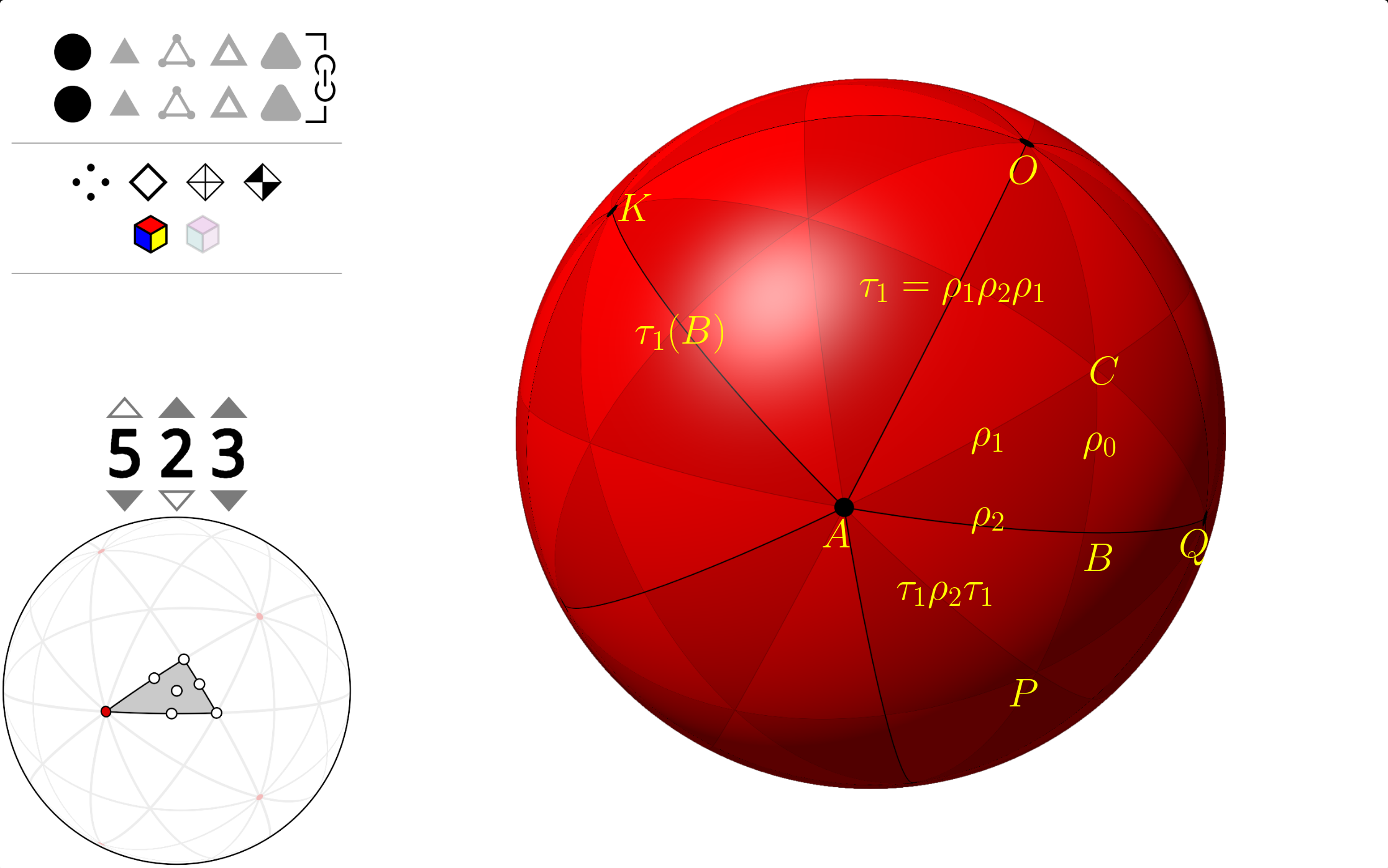You can see $\Delta ABC$ contains three copies of the fundamental domain of the icosaheron, so it covers the sphere three times under the symmetry group, this means it has "density" 3. If we continue to use $\rho_0,\rho_1,\rho_2$ denote the reflections about the mirrors $BC, AC, AB$，then these three reflections satisfy an extra relation which cannot be derived from their dihedral relations: let $D$ be the image of $B$ about the mirror $AC$, then the reflection about $AD$ is $\rho_1\rho_2\rho_1$ (we recall that if the reflection about mirror $\alpha$ is $s_\alpha$ and $g$ is an invertible linear transformation, then the reflection about mirror $\beta=g\alpha$ is $gs_\alpha g^{-1}$). So $\rho_0\rho_1\rho_2\rho_1$ is the composition of the reflections about $BC$ and $AD$, which is a rotation about the intersection of the two great circles that contain $BC$ and $AD$, which is $O$. You can see the angle between $BC$ and $AD$ is $\pi/3$, so the roration has angle $2\pi/3$, hence of order 3, hence $(\rho_0\rho_1\rho_2\rho_1)^3=1$.

This phenomena was observed by Coxeter in his article

Regular skew polyhedra in three and four dimensions, and their topological analogues.

Note all regular star 4d polytopes have the same symmetry group with the 120-cell $(5, 3, 3)$. Two more examples:

• The great grand stellated 120-cell $(5/2, 3, 3)$, it has a single extra relation $(\rho_1\rho_2\rho_3\rho_2)^3=1$:• The rectified version of grand stellated 120-cell $(5/2, 5, 5/2)$, this one has two extra relations $(\rho_0\rho_1\rho_2\rho_1)^3=(\rho_1\rho_2\rho_3\rho_2)^3=1$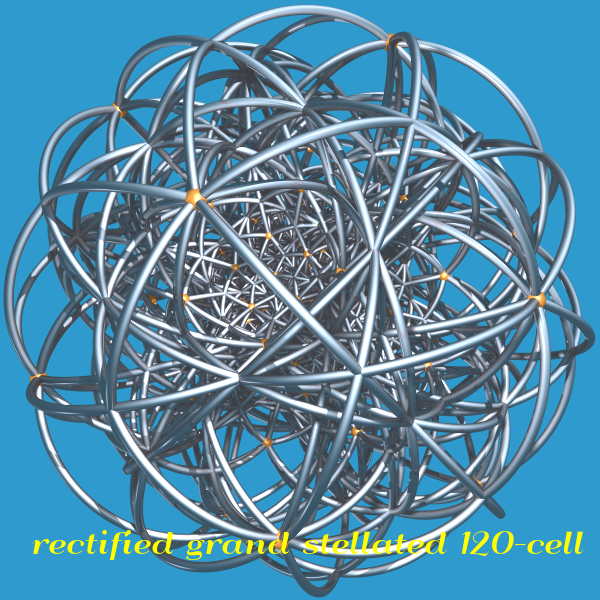# Appendix I

I also added a script run_coset_enumeration.py for showing how to compute the coset table of $G/H$ for a given finitely presented group $G$ and its subgroup $H$ (necessarily $|G/H|<\infty$). It assumes a yaml file as input which describes the presentation of $G$ and $H$. An example format is

Here we use the convention that uppercase means the inverse of lowercase, i.e. $A=a^{-1},B=b^{-1}$.

So the presentation of this group is $G = \langle a, b\ |\ a^8=b^7=(ab)^2=(a^{-1}b)^3=1\rangle$ and $H=\langle a^2, a^{-1}b\rangle$.

Save this file as G8723.yaml and run

The output should be (with some output omitted)

so $G/H$ has 448 cosets.

# Appendix II

Update: I made a raytraced image in POV-Ray to show Wythoff's construction, using the dodecahedron as example:The three mirrors $m_0,m_1,m_2$ are colored red, green and blue respectively. The "fundamental room" is the convex cone formed by these three mirrors, our initial vertex $v_0$ lies on the boundary of this room (the intersection axis of green and blue mirrors) and is colored magenta.